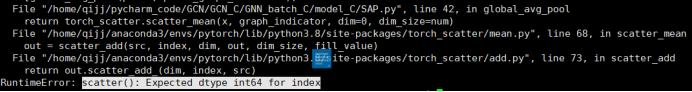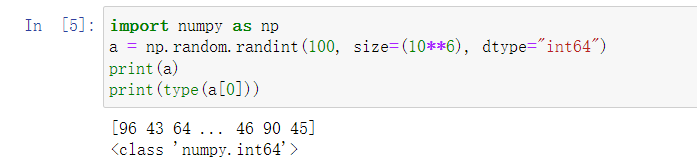# [Solved] RuntimeError: scatter(): Expected dtype int64 for indexRuntimeError: scatter(): Expected dtype int64 for index

1. Error reporting reason:

Scatter requires the data to be of type Int64, and I wrote torch when defining tensor Tensor (x) should be written as torch Longtensor (x), specified as Int64 type.

2. Solutions

Find the definition method of the original data and change it
generally, dtype = NP int64; dtype=np.
in float32 (most definition functions have dtype attribute)
it is better to have the same number of bits of int and float

``````import numpy as np
a = np.random.randint(100, size=(10**6), dtype="int64")
print(a)
print(type(a))
``````